Maths Brain Teasers 98 :: Can You - Decode The Logic to replace the Question Mark?Hey All; @StemGeeks Mathematician;

Without further, ado, let's get to our puzzle for today:: Maths Brain Teasers 98 :: Can You - Decode The Logic to replace the Question Mark?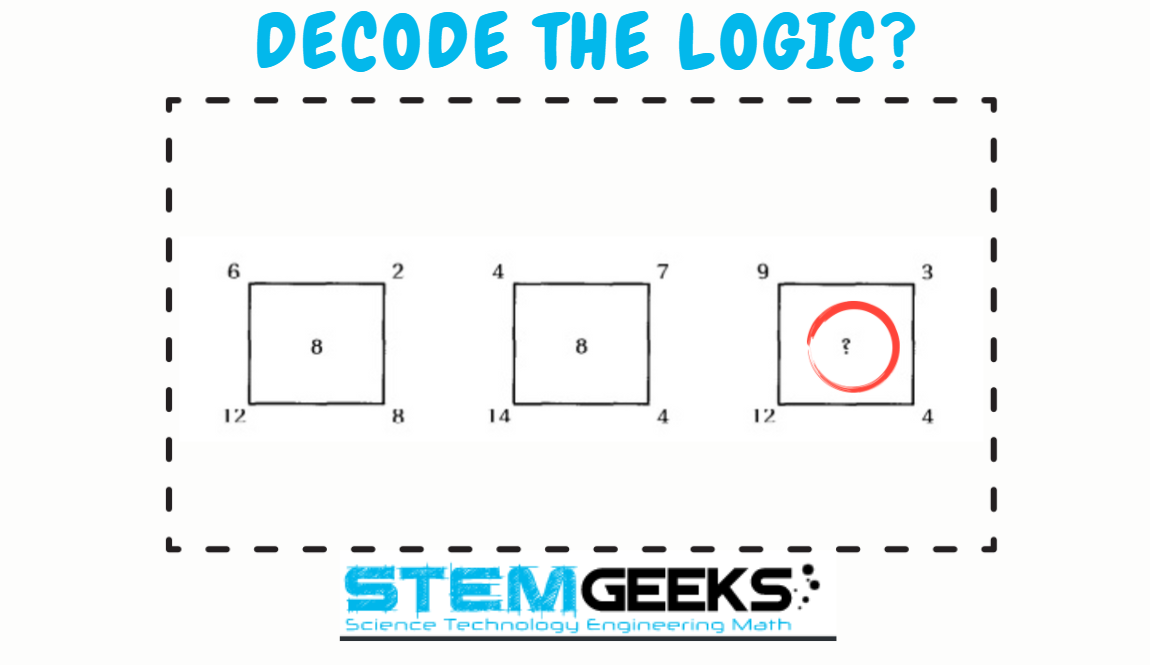Have a close look at the image given and see if you can decode the logic given in the image to complete the puzzle. Try finding out the right NUMBER that Simplifies or Decodes the logic to the given puzzle. There is logic to resolve the puzzle; try finding out the logic and it should be resolved in seconds.. Guesswork isn't going to help...

With that, I'll leave you all with the Maths Brain Teasers 98 :: Can You - Decode The Logic to replace the Question Mark? Good luck solving the puzzle...

Maths Brain Teasers 97 :: Can You - Complete The Circle? - Solved with Explanation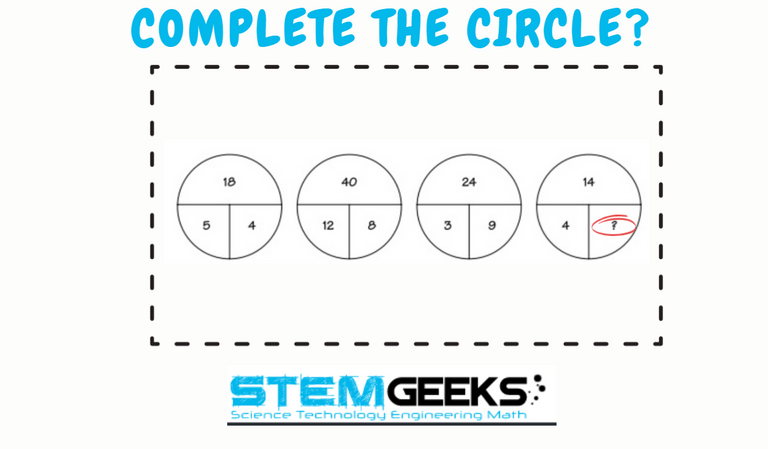Let's have a look at the Step by Step instructions to Solve the Maths Brain Teasers 97 :: Can You - Complete The Circle?

If you carefully look at the Image in question; You would have noticed the following pattern is being followed::

The logic was::

First, we do the sum of the bottom two numbers in each circle first. Then double that number, which would represent the number of the top of the circle.

Let's take the example 1::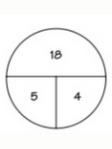5 + 4 = 9 * 2 = 18

Now let's take the circle in question::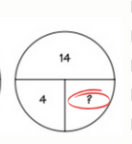4 + 3 = 7 * 2 = 14

Hence the answer to the Puzzle is Number:: 3

STEM token GiveAway

I'll be again doing a giveaway of STEM tokens to the lucky random winner with the correct answer. For the last contest, which was Maths Brain Teasers 97 :: Can You - Complete The Circle?.

We had 3 entries and I am happy to see the engagement by everyone. At the same time, it's great to see the detailed answers provided by everyone as to how they arrived at the solution of the puzzle.. Well done guys.. Way to GO... Now the results part; all the entries provided the right answer. So, I am going to split the rewards between them.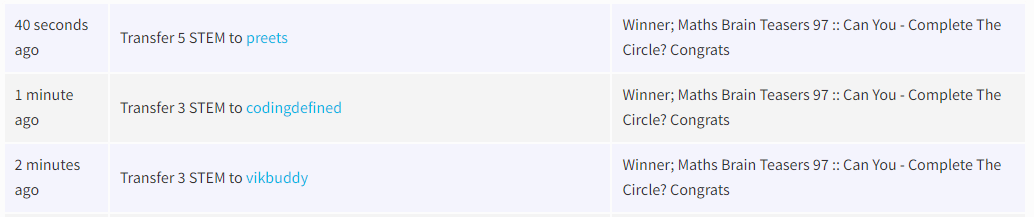Congratulations @vikbuddy, @preets && @codingdefined - You WIN yourself 3 STEM tokens. You should be having the rewards in your STEM Wallet Soon.

Math Quote for the Day::

Here is the motivation to solve the Maths Equation Puzzle?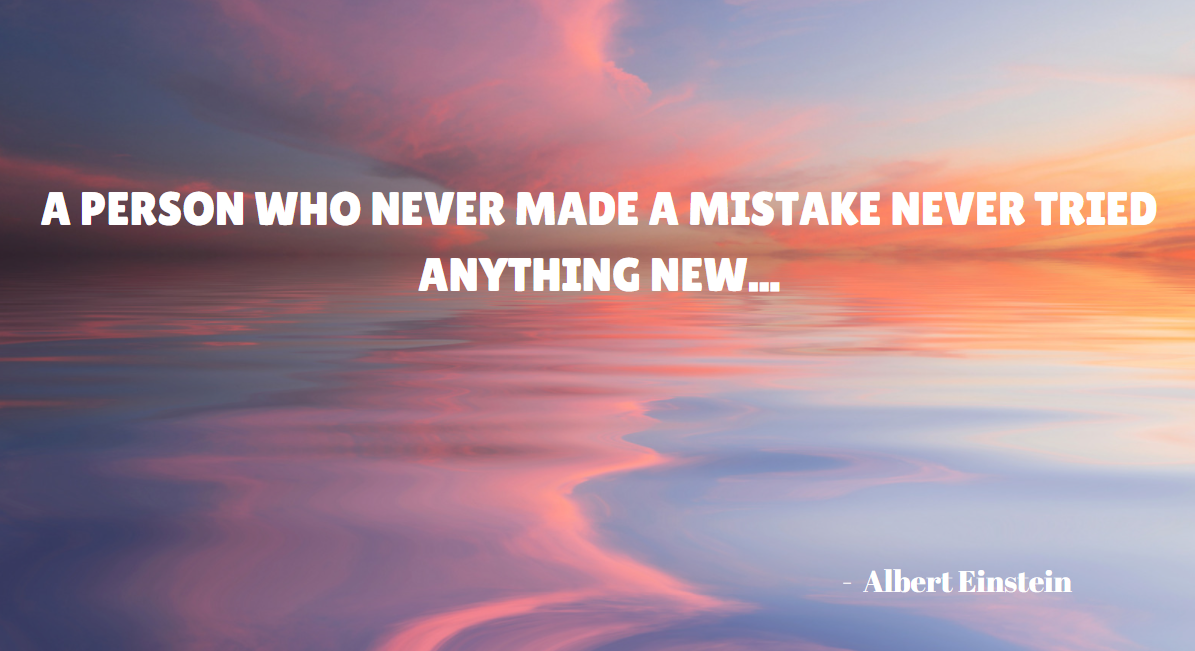If you like my work, then please spread the Word.. that we do have the Math Brain Teasers competition here @StemGeeks platform. Reblog is much appreciated.

Best RegardsFind Me on the Other Social Media Platforms::PS:: All the Maths Brain Teasers; are made by me using the Pro Canva License Version

0
0
0.000